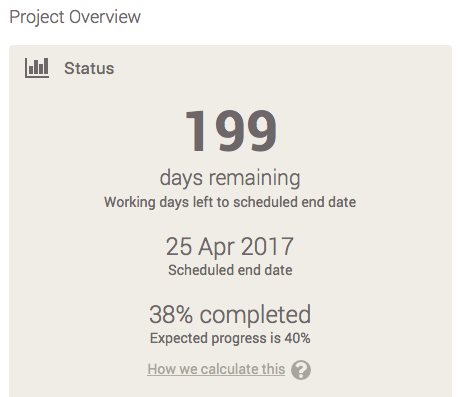# Calculation of Project Progress Percent

You can see the calculated project completion from the dashboard, next to the project title, and from the overview screen. The expected progress is also calculated on the overview screen.Percent Completed

First, we calculate the total number of working days of the project by adding the duration of each task together, not including non-working day, giving the Total Number of Working Days.

For example, if you have 3 tasks, each 4 days duration, the Total Number of Working days will be 12.

Second, we look at the progress percentage for each task and convert it into number of working days completed. We then add all these working days together, not including non-working days, giving the Total Number of Working Days Completed.

For example, a 4 day task with 50% complete, will give 2 working days completed.

To get the Percent Completed, we divide the Total Number of Working Days Completed by the Total Number of Working Days.

Expected progress

To get the Expected Progress we look at Todays Date Line within the Gantt, and for each task we calculate what percentage of the Task should be complete by Todays Date Line. This percentage is then converted into Number of Working Days Expected Complete. We then add all these together for each tasks giving a Total Number of Working Days Expected Complete.

For example, if Today is 2 days into a 4 day task, we would expect 50% to be complete, giving 2 Working Days Expected Complete. If a task has not started we would expect 0% complete. If an end date is before today, we would expect 100%.

To get Expected Progress, we divide Total Number of Working Days Expected Complete by the Total Number of Working Days.